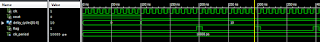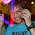VHDL coding tips and tricks: A synthesizable delay generator instead of 'wait for' statement

## Thursday, March 4, 2010

### A synthesizable delay generator instead of 'wait for' statement

CODE UPDATED ON 01/11/2017 AFTER IMPROVISING!

There are many situations in which you may need to activate a process after a certain delay or at fixed time intervals.If you want to do simulation alone for your design then you can simply use "wait for" statement to call a delay routine.But this keyword is not synthesizable. So what will you do in such situations?

A simple delay routine,which is synthesizable, can be designed using the properties of a "MOD-n counter". A MOD-n counter can be used to generate a frequency of (f / n) using a frequency 'f'. The code for generating such a delay is given below:

VHDL Design:

library IEEE;
use IEEE.STD_LOGIC_1164.ALL;
use IEEE.NUMERIC_STD.ALL;

entity delay_gen is
port (clk,reset : in std_logic;
delay_cycles : in unsigned(31 downto 0);   --delay to be generated.
flag : out std_logic --this is a pulse to notify that time interval equal to delay is over.
);
end delay_gen;

architecture Behavioral of delay_gen is

signal count : unsigned(31 downto 0) := (others => '0');

begin

process(clk,reset)
begin
if(reset = '1') then
flag <= '0';
count <= (others => '0');
elsif(rising_edge(clk)) then
--when the necessary delay is achieved, reset count and set flag high
if(count = delay_cycles-1) then
count <= (others => '0');
flag <= '1';
else
flag <= '0';
count <= count +1;   --increment counter otherwise.
end if;
end if;

end process;

end Behavioral;

The module has 3 inputs including a clock and a reset signal. The delay_cycles input determines the amount of delay generated by the code.

We can write,

delay_cycles = [Delay in seconds] / [Clock period of clk].

Say you want a 100 ns delay. Now say your clk frequency is 100 MHz. That  means your clock period is 10 ns. So the value of delay_cycles should be equal to (100 ns / 10 ns) = 10. When the counter counts from 0 to 9, it sends a pulse to notify.

I have written a testbench code, to show how you can instantiate this module.

Testbench Code:

LIBRARY ieee;
USE ieee.std_logic_1164.ALL;
USE ieee.numeric_std.ALL;

ENTITY tb IS
END tb;

ARCHITECTURE behavior OF tb IS

-- Component Declaration for the Unit Under Test (UUT)
COMPONENT delay_gen
port (clk,reset : in std_logic;
delay_cycles : in unsigned(31 downto 0);   --delay to be generated.
flag : out std_logic --this is a pulse to notify that time interval equal to delay is over.
);
END COMPONENT;

--Inputs
signal clk : std_logic := '0';
signal reset : std_logic := '0';
signal delay_cycles : unsigned(31 downto 0) := (others => '0');
--Outputs
signal flag : std_logic;

-- Clock period definitions
constant clk_period : time := 10 ns;

BEGIN

-- Instantiate the Unit Under Test (UUT)
uut: delay_gen PORT MAP (
clk => clk,
reset => reset,
delay_cycles => delay_cycles,
flag => flag
);

-- Clock process definitions
clk_process :process
begin
clk <= '1';
wait for clk_period/2;
clk <= '0';
wait for clk_period/2;
end process;

-- Stimulus process
stim_proc: process
begin
reset <= '1';
wait for clk_period*10;
reset <= '0';
--by setting the input as "10", I want a delay of 10 clock cycles.
--in real time,the delay = 10*period of clock.
--so here in simulation, delay = 10*10 ns = 100 ns.
delay_cycles <= to_unsigned(10,32);
wait;
end process;

END;

Simulation Waveform:This small code can be used under many situations,like :

1. To run some parts of your design at a lesser clock frequency than your system clock.
2. To create a delay between some of the processes.

The range of delay values can be increased by increasing the size of delay_cycles. Also the maximum error in the delay produced is equal to the period of input clock.

#### 11 comments:

1.Thanks for the code,

Can I have the same in verilog?

2.@kartik : yeah. You can use the basic idea here to write a similar code in verilog.

3.but how do i use this in a desgin code? for example if i want to implement a flip flop how do i encode this mod-n counter in the code for flip flop...can u please give an example? I'm a novice.

4.@nebula : any MOD-n counter can be designed using flip flops.You just have to reset all the flip flops when the output reaches the particular count.If it is 3 bit counter , and you want the max count to be "5" then the reset input of all FF's should be connected to R= count(0) xor (not count(1)) xor count(2).

5.Hi,

Is there any other way other than a counter to make the delay synthesizable.

Suppose if dealy to be introduced is 8.114ns, then how to add a delay element for 8.114ns and it has to be synthesizable.

6.I get a warning "line 18: One or more signals are missing in the process sensitivity list. To enable synthesis of FPGA/CPLD hardware, XST will assume that all necessary signals are present in the sensitivity list. Please note that the result of the synthesis may differ from the initial design specification. The missing signals are:
"
and I dont get output if I add count to the sensitivity list....
what shall I do?

7.how to run 2nd part (---- a program which uses a delay routine of 100 ns.)in vhdl??
I mean can some one help me out to make the above code run with both upper and lower part..

8.Hey,

I need a code for a delay of about 30 seconds to 30 minutes using a clock of 50 MHz.
The above program doesnt seem to work.

9.The code looks wrong. It will probably work in simulation but not on the real hardware. The first process is missing "count" in the sensitivity list. That's why the simulation works. If you add count to the sensitivity list you will notice that the simulation produces a very short pulse (much shorter than a clock cycle). You have to move the first "end if;" in that process to the end of the process and it will work.

Second thing is that the other process uses this output from the first one as a clock signal. This will most likely work for simple cases, but can lead to nasty problems because it isn't routed on a clock network. So instead you should use it as an enable signal:

process(clk)
begin
if(clk'event and clk='1') then
if (flag='1') then
output <= calculated; -- this line is executed only once in 100 ns.
end if;
end if;
end process;

As a rule of thumb if you have and other signal than "clk" in your sensitivity list and you aren't absolutely sure why you need it you are doing it work.

10.How can i generate a signal with delay of 50ns when low,5 us when high.
M actually trying to interface an ad7608 with fpga..

11.Hi
thank you for sharing the code.
I modified the code little bit. I am using Spartan-6 FPGA.My board contains 50 MHz Clock.That means my clock period should be 20 ns. So the value of delay should be equal to (5000000000 ns / 20 ns) = 250000000. So, when the counter counts from 0 to 249000000 the led should light up.Below is the program. But it is not working as i intended it to work.Can you please help to rectify the issue with the program.
----------------------------------------------------------------------------------------------------
library IEEE;
use IEEE.STD_LOGIC_1164.ALL;
use IEEE.NUMERIC_STD.ALL;

entity test123 is
port (clk,reset : in std_logic;
led : out std_logic
);
end test123;

architecture Behavioral of test123 is

signal count : unsigned(27 downto 0) := (others => '0');

begin

process(clk,reset)
begin
if(reset = '0') then
led <= '0';
count <= (others => '0');
elsif(rising_edge(clk)) then
if(count = 250000000-1) then
count <= (others => '0');
led <= '1';
else
led <= '0';
count <= count +1; --increment counter otherwise.
end if;
end if;

end process;
end Behavioral;